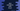# Java program to print from A to Z## Java program to print A to Z:

In this post, we will learn how to print from A to Z in Java. I will show you different ways to do the printing. With this post, you will learn how loops work in Java.

### Example 1: Java program to print A to Z by using a for loop:

We can iterate through A to Z with a loop. Instead of an integer variable, we have to use a character variable in the loop.

``````public class Main {
public static void main(String[] args) {
for (char c = 'A'; c <= 'Z'; c++) {
System.out.print(c + " ");
}
}
}``````

In this post, we are using a for loop that runs from c = ‘A’ to c = ‘Z’ and on each iteration, it increments the value of c by 1. These are characters but since the ASCII values of A to Z are from 65 to 90, the loop iterates over these values and on each increment, it moves to the next character.

It will print the alphabet:

``A B C D E F G H I J K L M N O P Q R S T U V W X Y Z``

We can use the same approach to print the alphabet in lowercase:

``````public class Main {
public static void main(String[] args) {
for (char c = 'a'; c <= 'z'; c++) {
System.out.print(c + " ");
}
}
}``````

This is similar to the above example. The only difference is that we are looping through 97 to 122 in this example.

It will print:

``a b c d e f g h i j k l m n o p q r s t u v w x y z``

### Example 2: Java program to print A to Z by using a while loop:

Let’s use a while loop to print from A to Z:

``````public class Main {
public static void main(String[] args) {
char c = 'A';
while (c <= 'Z') {
System.out.print(c + " ");
c++;
}
}
}``````

In this approach, we have to initialize the value of c before the loop starts. The value of c is printed inside the loop and at the end of each iteration, its value is incremented by 1.

It will give similar results.

### Example 3: By using a do-while loop:

The do…while loop works similarly to a while loop. It executes the code written inside the do block and then checks the condition in the while block.

``````public class Main {
public static void main(String[] args) {
char c = 'A';
do {
System.out.print(c + " ");
c++;
} while (c <= 'Z');
}
}``````